Courses

# Concepts of Mass, Weight, Thrust, Pressure and Density Class 9 Notes | EduRev

## Class 9 : Concepts of Mass, Weight, Thrust, Pressure and Density Class 9 Notes | EduRev

The document Concepts of Mass, Weight, Thrust, Pressure and Density Class 9 Notes | EduRev is a part of the Class 9 Course Science Class 9.
All you need of Class 9 at this link: Class 9

MASS

The amount of matter contained in a body is called its mass
or
The measure of the quantity of matter In a body is called its mass.
The mass of a body is a scalar quantity. It is independent of surroundings and theposition of the body. It is a constant quantity for a given body.

Mass is measured in kilograms (kg) in SI system.

Characteristics of mass of a body :-
1. Mass of a body is proportional to the quantity of matter contained in it.
2. Mass of a body does not depend on the shape, size and the state of the body.
3. Mass of a body remains the same at all place. This means, the mass of a body will be same throughour the universe. This is because the quantity of matter contained in the body does not change throughout the universe.
4. Mass of a body does not change in the presence of other bodies near it.
5. Mass of a body is a scalar quanity.
6. Mass of a body can be measured with the help of a beam balance.
7. Masses of object or bodies are added algebrically.

Weight

The force with which a body is attracted by the earth is known as the weight of the body.
When the earth attracts a body with a gravitational force, the body accelerates towards the earth with an acceleration due to gravity (g).
Thus, the force with which body of mass m is attracted by the earth is given by
F = ma = m × g = mg
This force is known as the weight of the body. Weight of a body is denoted by W.

∴ Weight, W = mg
Weight has both magnitude and direction. Hence weight is a vector quantity.

Unit of Weight :-
SI unit of weight is same as that of the force i.e., newton (N).

Variation in the weight of a body
Weight of the body is given by
W = mg
So the weight of a body depends upon (i) the mass of the body and (ii) value of acceleration due to gravity.

The mass of a body remains the same throughout the universe, but the value of 'g' is different places. Hence the weight of a body is different at different place.
1. The value of 'g' is more at poles and less at the equator. Therefore, weight of a body is more at the poles and less at the equator. In other words, a body weighs more at the poles and less at the equator.
2. The value of 'g' on the surfaces of different planets of the solar system is different, therefore, the weight of a body is different on the different planets.
3. The value of 'g' decreases with height from the surface of the earth. Therefore, the weight of a body also decreases with height from the surface of the earth. That is why, the weight of a man is less on the peak of Mount Everest than the weight of the man at Delhi.
4. The value of 'g' decreases with depth from the surface of the earth. Therefore, the weight of a body decreases with depth from the surface of the earth.
5. The value of 'g' at the centre of the earth is zero, hence weight (= mg) of the body is zero at the centre of the earth.

 Difference Between Mass and Weight S. No. Mass Weight 1 The quantity of matter contained in a body is called the mass of the body. The force with which the earth attracts a body towards its centre is called the weight of the body. 2 Mass of a body remains constant. Weight of a body changes from place to place. 3 Mass of a body is never zero. Weight of a body at the centre of the earth is zero. 4 Mass is scalar quantity. Weight is a vector quantity. 5 Mass is a measured in kg. Weight is measured in kg wt or N. 6 Mass is measured by a beam balance. Weight is measured by a weighing machine or a spring balance.

PRACTICAL UNITS of weight
In SI, the weight is also measured in kg f or kg wt.
Therefore, kilogram force or kilogram weight is force with which a mass of 1 kg is attracted by centre of earth.
1 kg f = 1 kg wt = 1 kg × 9.8 m/s2 = 9.8 kg ms-2 = 9.8 N
1 kg F = 9.8 N
In CGS, the practical unit of weight is grams force or g wt or 1g f or 1g wt is force with which a mass of 1g is attracted by the centre of the earth.
g = 9.8 ms-2
g = 9.8 × 100 cm/s2
1 g f = 1 g wt = 1 g × 980 cm s-2 = 980 dyne
1 g f = 980 dyne

Thrust and pressure

Thrust : Force acting normally on a surface is called the thrust.
Thrust is a vector quantity and is measured in the unit of force, i.e., newton (N).
Pressure : The thrust acting on unit area of the surface is called the pressure.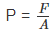If a thrust F acts on a area A, then pressure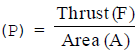Pressure is directly proportional to the force.
Pressure in inversely proportional to the area.

For the examples :
Ex. A sharp knife cuts easily than a blunt knife by applying the same force.
Ex. A sharp needle pressed against our skin pierces it. But a blunt object with a wider contact area does not affect the skin when pressed against it with the same force.
Some interesting aspects of pressure
1. The foundation of a building or a dam has a large surface area so that the pressure exerted by it on the ground is less. This is done to prevent the sinking of the building or the dam into the ground.
2. The tyres of a bus or a truck have larger width than those of a car. Further, the number of tyres of heavy vehicles is more than four. This is done to enable the tyres to carry more weight and to prevent sinking

into ground.|

3. A sleeping mattress is so designed that when you lie on it, a large area of your body comes in its contact. This reduces the pressure on the body and sleeping becomes comfortable.
4. Railway track are laid on large sized wooden or iron sleepers.
We know, Pressure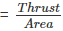The weight (i.e., thrust) of the train is spread over a large area of the sleepers. Therefore, the pressure acting on the ground under the sleepers is reduced. This prevents the sinking of the ground under the weight of the train.

5. A sharp knife is more effective in cutting the objects than a blunt knife.
The pressure exerted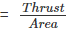The area under the shrap knife is less than the area under the blunt knife. Hence, the pressure exerted by the sharp knife is more than the pressure exerted by the blunt knife on an object. Therefore, the sharp knife penetrates easily into the object than the blunt knife when same force is applied in both the cases. Hence, a sharp knife cuts the objects easily than a blunt knife.

6. A camel walks easily on the sandy surface than a man inspite of the fact that a camel is much heavier than a man.
This is because the area of camel's feet is large as compared to the area of man's feet. So the pressure exerted by camel on the sandy surface is very small as compared to the pressure exerted by man. Due to large pressure, sand under the feet of a man yields (i.e. sink) and hence he cannot walk easily on the sandy surface.

7. A sharp needle, pierce the skin easily but not a blunt needle although the force applied on both the needles is same.

Pressure exertedThe area under the pointed end of the sharp needle is very small as compared to the area under the pointed end of the blunt needle. So pressure exerted by the sharp needle is much more than the pressure exerted by the blunt needle. Hence a sharp needle pierces the skin easily than the blunt needle.

8. It is painful to hold a heavy bag having strap made of a strong and thin string.
We know, PressureWhen we hold a heavy bag having strap made of a strong and thin string, then the are under the strap is small. Hence, large pressure is exerted by the strap on our fingers or shoulder. Due to this large pressure, the strap tends to cut the skin and hence pain is caused.

9. The army tank has a large weight. Therefore to avoid large pressure on the ground its weight is distributed throughout the tank. This is done by making the tank run on a steel track rather than on wheels. The steel tracks reduce the pressure of the ground .

Units of Pressure. The SI unit of pressure is called pascal (Pa) in honour of Blaise Pascal.
I Pa = 1 N/m2

One pascal is defined as the pressure exerted on a surface area of 1 m2 by a thrust of 1 N (acting normally on it).
Other units of pressure are bar and millibar where
1 bar = 105 N/m2 and 1 millibar = 102 N/m2
It is a common practice in meteorology to measure atmospheric pressure in bars and millibars. Further,
1 atmospheric pressure (1 atm) = 101·3 k Pa = 1·013 bar = 1013 m bar

Density
Density of a substance is defined as its mass per unit volume.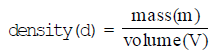Unit of density :- Since mass (M) is measured in kilogram (kg) and the volume (V) is measured in metre3 (m3),
the unit of density is kg/m3. In cgs system, the unit of density is g cm–3.

These units are related as : 1 g cm–3 = 1000 kgm–3.

RELATIVE DENSITY

Relative density (R.D.) of a substance is the ratio of the density of the substance to the density of water at 4°C.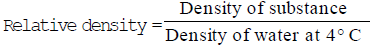Unit of Relative Density
Since relative density is a ratio of two similar quantities, it has no unit.
Further,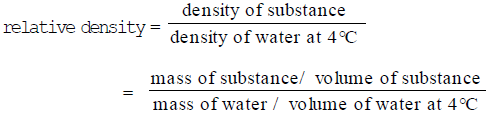If the volume of a given substance is equal to the volume of water at 4°C,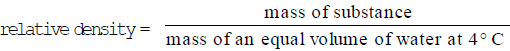Relative density can also be defined as the ratio between the mass of the substance and the mass of an equal volume of water at 4°C.

Offer running on EduRev: Apply code STAYHOME200 to get INR 200 off on our premium plan EduRev Infinity!

## Science Class 9

95 videos|223 docs|135 tests

,

,

,

,

,

,

,

,

,

,

,

,

,

,

,

,

,

,

,

,

,

,

,

,

,

,

,

,

,

,

;